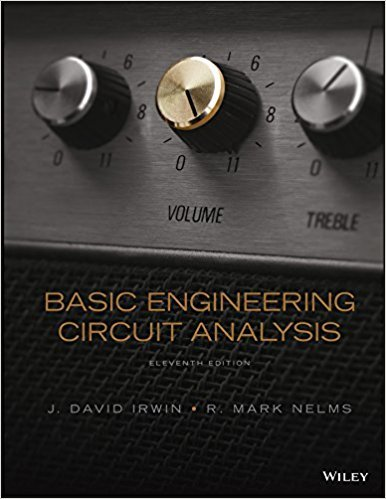×
Get Full Access to Basic Engineering Circuit Analysis - 11 Edition - Chapter 6 - Problem 6.49
Get Full Access to Basic Engineering Circuit Analysis - 11 Edition - Chapter 6 - Problem 6.49

×

# Determine the value of C T in the circuit in Fig. P6.49. 5ISBN: 9781118539293 159

## Solution for problem 6.49 Chapter 6

Basic Engineering Circuit Analysis | 11th Edition

• Textbook Solutions
• 2901 Step-by-step solutions solved by professors and subject experts
• Get 24/7 help from StudySoup virtual teaching assistantsBasic Engineering Circuit Analysis | 11th Edition

4 5 1 410 Reviews
11
0
Problem 6.49

Determine the value of C T in the circuit in Fig. P6.49. 5 F 6 F 6 F 12 F 11 F 9 F 3 F CT Figure P6.49

Step-by-Step Solution:
Step 1 of 3

E Theory Notes--08/24/16 22 August, 2016 07:55 AM E Theory Page 1 E Theory Page 2 E Theory Page 3

Step 2 of 3

Step 3 of 3

##### ISBN: 9781118539293

The full step-by-step solution to problem: 6.49 from chapter: 6 was answered by , our top Engineering and Tech solution expert on 11/23/17, 05:00AM. Since the solution to 6.49 from 6 chapter was answered, more than 284 students have viewed the full step-by-step answer. This textbook survival guide was created for the textbook: Basic Engineering Circuit Analysis, edition: 11. Basic Engineering Circuit Analysis was written by and is associated to the ISBN: 9781118539293. The answer to “Determine the value of C T in the circuit in Fig. P6.49. 5 F 6 F 6 F 12 F 11 F 9 F 3 F CT Figure P6.49” is broken down into a number of easy to follow steps, and 29 words. This full solution covers the following key subjects: Circuit, determine, fig, figure. This expansive textbook survival guide covers 15 chapters, and 1430 solutions.

Unlock Textbook Solution